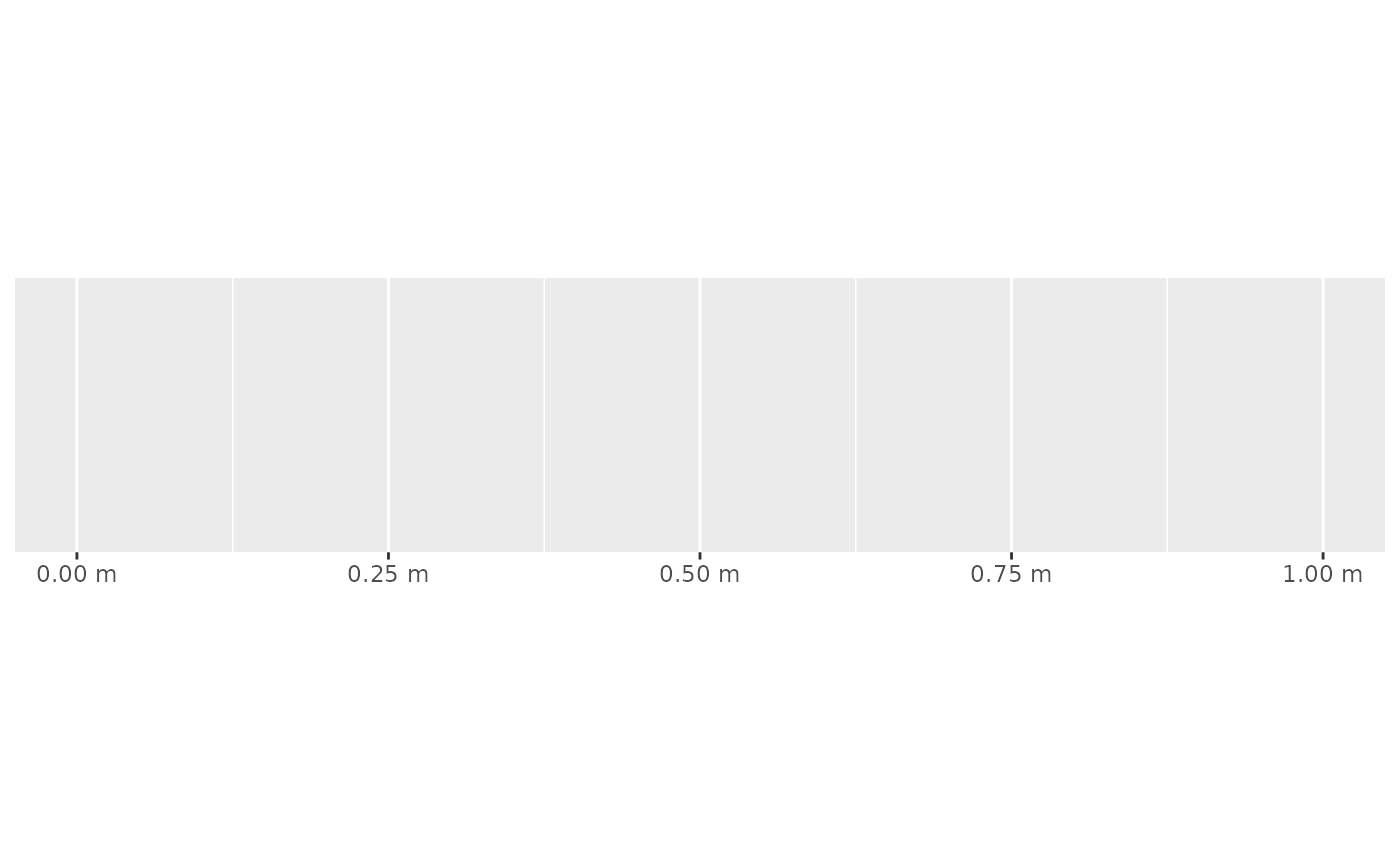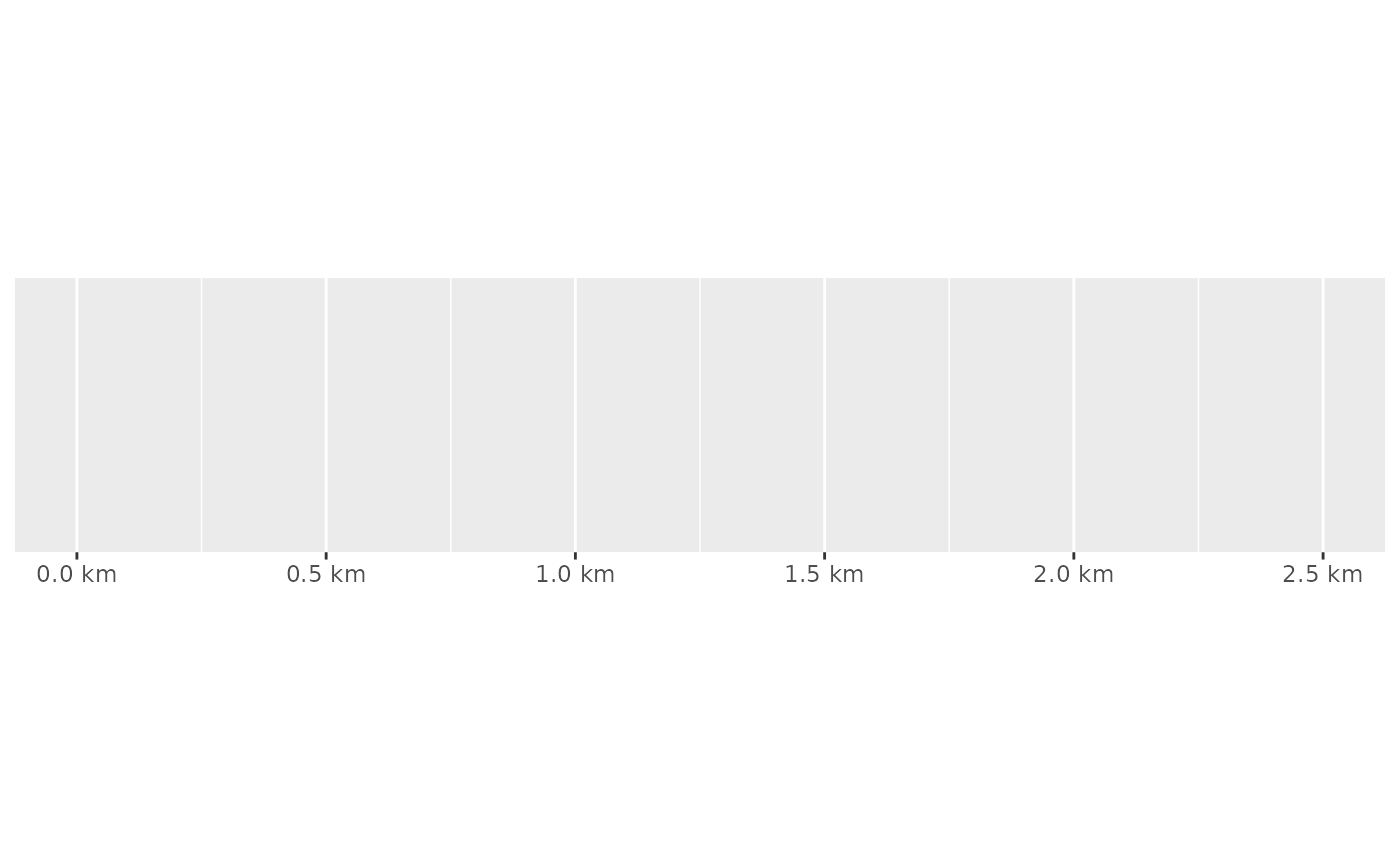This function is kept for backward compatiblity; you should either use label_number() or label_number_si() instead.

## Usage

unit_format(
accuracy = NULL,
scale = 1,
prefix = "",
unit = "m",
sep = " ",
suffix = paste0(sep, unit),
big.mark = " ",
decimal.mark = ".",
trim = TRUE,
...
)

## Arguments

accuracy

A number to round to. Use (e.g.) 0.01 to show 2 decimal places of precision. If NULL, the default, uses a heuristic that should ensure breaks have the minimum number of digits needed to show the difference between adjacent values.

Applied to rescaled data.

scale

A scaling factor: x will be multiplied by scale before formatting. This is useful if the underlying data is very small or very large.

prefix

Additional text to display before the number. The suffix is applied to absolute value before style_positive and style_negative are processed so that prefix = "$" will yield (e.g.) -$1 and (\$1).

unit

The units to append.

sep

The separator between the number and the unit label.

suffix

Additional text to display after the number.

big.mark

Character used between every 3 digits to separate thousands.

decimal.mark

The character to be used to indicate the numeric decimal point.

trim

Logical, if FALSE, values are right-justified to a common width (see base::format()).

...

Other arguments passed on to base::format().

## Examples

# Label with units
demo_continuous(c(0, 1), labels = unit_format(unit = "m"))
#> scale_x_continuous(labels = unit_format(unit = "m"))# Labels in kg, but original data in g
km <- unit_format(unit = "km", scale = 1e-3, digits = 2)
demo_continuous(c(0, 2500), labels = km)
#> scale_x_continuous(labels = km)